﻿ Digital Circuits and Systems - Circuits i Sistemes Digitals (CSD) - EETAC - UPCBachelor's Degree in Telecommunications Systems and in Network Engineering## Synchronous 1-digit BCD counter

Plan Y: FSM using STD_LOGIC_VECTOR and arithmetic libraries

1. Specifications

Design a synchronous 1-digit BCD counter following a FSM strategy where all the states will be enumerated. The chip will include an asynchronous reset (CD) and also a count enable (CE) input and a terminal count (TC10) output to allow expandability.Fig. 1. Symbol and function table. You can also draw an example timing diagram for this circuit.

It is a good idea to read a typical datasheet of a classic chip that has similar features, like the 74LS162. And it is also necesary to draw an example sketch of timing diagram to be able to apply the function table.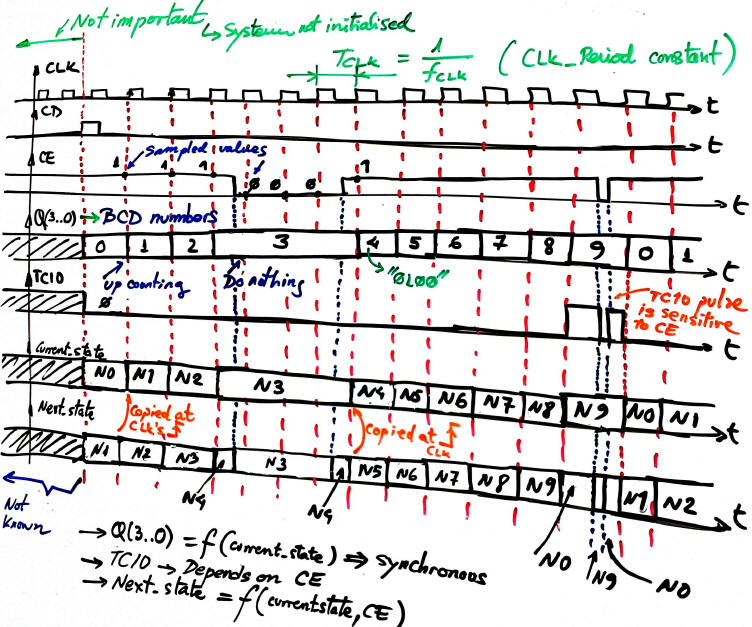Fig. 2. An example timing diagram for this counter. Solve it in a sheet of paper annotating on the waveforms all the important details over time. You can also represent the evolution of the internal FSM signals current_state and next_state. The VHDL testbench functional simulation in the 4th design step is based on the translation of a diagram like this one.

The circuit in Fig. 3 is the complete example project solved using the Lattice ispLEVER Classic and Proteus ISIS so that the counter can be downloaded into a simple sPLD GAL22V10 target chip. In order to run it, download and unzip the compressed file in a given folder. The GAL symbol is connected to the binary configuration file counter_BCD_1digit_prj.jed where the sPLD fuses are programmed.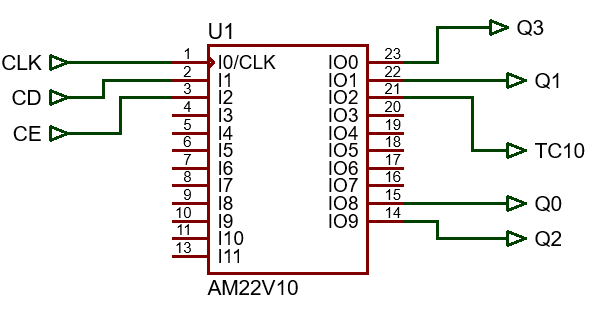Fig. 3. Schematic and pinning for the counter when targeting a sPLD GAL22V10.

2. Planning

Let's apply the methodology o build the counter. Thus, as you have seen in the tutorials, we'll follow always the same methodology to improve understanding and the reliability of the circuits designed

1) Figure out the state diagram. Number of states and conditions of transitions. Outputs at each state. In this example, there are 10 states and 20 state transitions.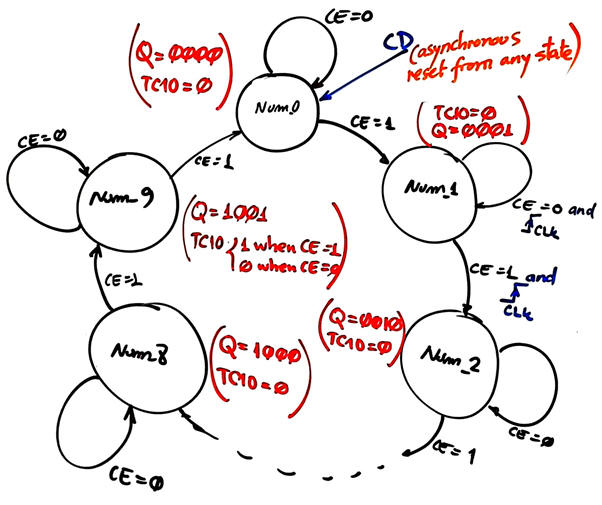Fig. 4. State diagram where the arrows indicate the transitions between states accordingly to the given condition, and in parenthesis in each state there is the indication of the output values. To make it easy, different colours are used to represent outputs and transitions. 20 transitions, 10 states, 20 transitions.

2) Customise the general FSM topology to this problem indicating where all the inputs and outputs are connected. The number of bits r for coding in binary sequencial this modulo 10 counter is r = 4.Fig. 5. The general topology of a finite state machine (FSM) particularised for this problem.

3) State register. We'll use always the same VHDL code description, which corresponds to the process of an r-bit D_FF with asynchronous reset. Draw the state register schematic.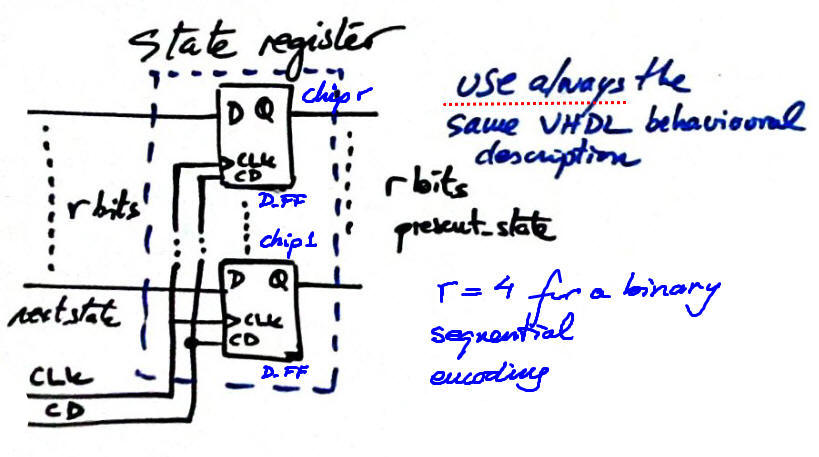Fig. 6. Sketch of the state register memory circuit, r D_FF working synchronously in parellel.

4) CC2 in Fig.7. Truth table and its behavioural description as a flowchart (plan B). The synthesiser will generate the final circuit from this process. The CC2 is required to calculate the outputs at each state. The outputs in the state diagram are annotated in parenthesis and in a different colour. In this application Q is simply a buffer copy of the internal current state.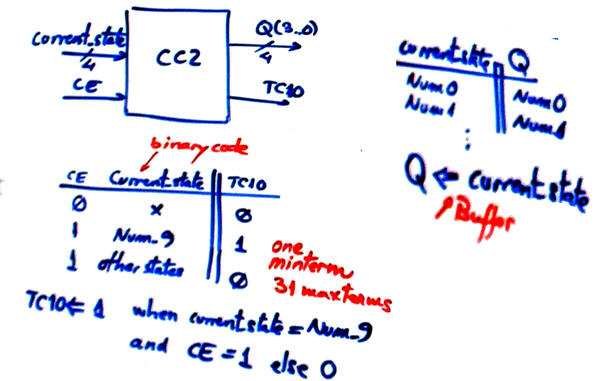Fig. 7. The truth table of the blocks CC2 and its equivalent flochart. CC2 generates all the outputs represented in parenthesis in each state.

5) CC1 in Fig.8. Truth table and its behavioural description as a flowchart (plan B). The synthesiser will generate the final circuit from this process. CC1 is required to calculate the next state to go after the CLK's rising edge.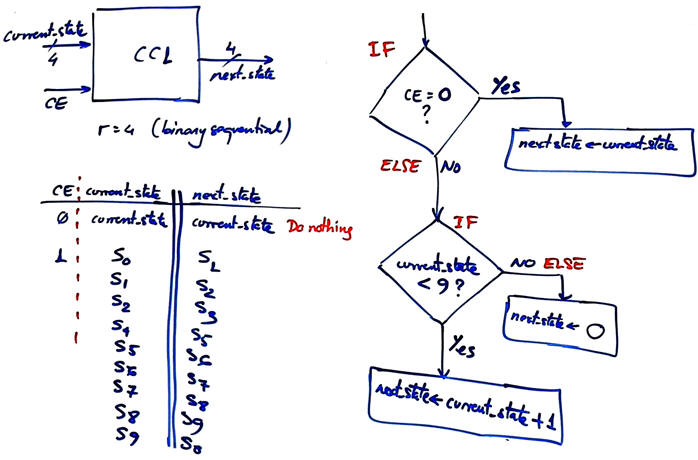Fig. 8. The truth table of the blocks CC1 and its equivalent flochart for executing all the state transitions represented as arrows in the state diagram.

6) The project name Counter_BCD_1digit_prj and folder location to develop the product:

L:/CSD/P7/Counter_BCD_1digit_Y/(files)

3. Development

Write down the VHDL file corresponding the the counter after having translated the flow charts of the CC1 and CC2 and copied the state register. Copy and adapt, for instance, this Counter_mod_12.vhd.

This is the Counter_BCD_1digit.vhd as translated from Fig. 6, 7 and 8 and using and adapting the file above. Run the EDA tool to synthesise the circuit and print and comment the RTL schematic using coloured pens. Can you identity each block? Check the number of registers (D_FF) used in the target chip.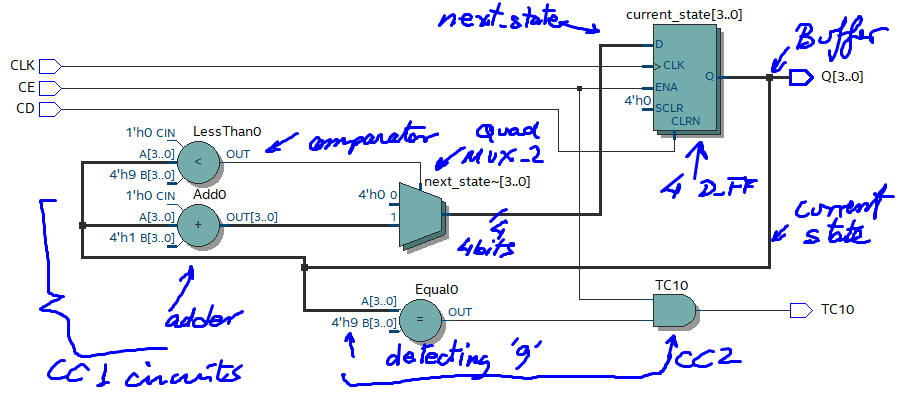Fig. 9. The RTL view using the EDA tool Quartus Prime from Intel.

Picture 10 shows the circuit in technology view.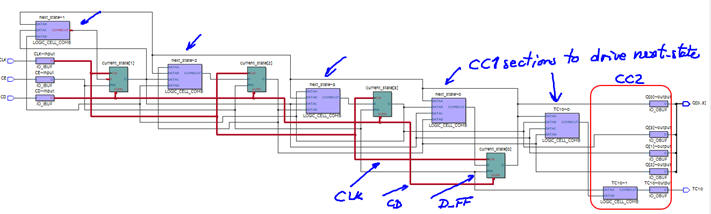Fig. 10. Technology view for a target chip Ciclone IV using EDA tools from Intel.

4. Testing (functional simulation)

Start the test bench template and add the CLK and inputs activity translating the timing diagram. Thus, to test sequential systems at least two stimulus processes will be required: the CLK and all the other inputs. Make all the timing relative to the CLK_Period constant because this parameter represents the time resolution of the digital system. If a signal is shorter than the CLK period it can not be seen (sampled) or detected (it's a glitch). Consequently, higher time resolutions implies higher CLK frequencies.Fig. 11. The test bench setup showing both, the CLK and other signals stimulus processes.

This is a sample test bench file counter_BCD_1digit_tb.vhd from which you can copy & adapt the input vectors.

Functional simulation. Run the EDA VHDL tool and demonstrate how the circuit works adding comments to the printed sheet of paper containing the waveforms.Fig. 12. Example of a  timing diagram to show how the counter works.

5. Testing (gate-level/timed simulations)

Gate-level simulation. Run the EDA VHDL tool using the same test bench and demonstrate the CLK to output delays and the maximum frequency of operation depending on the technology of the programmable chip use as the target device.Fig. 13. Example of a timing diagram to show how there is a propagation time CLK to output (tco) fora given target chip (Intel MAX II EPM2210F324C3).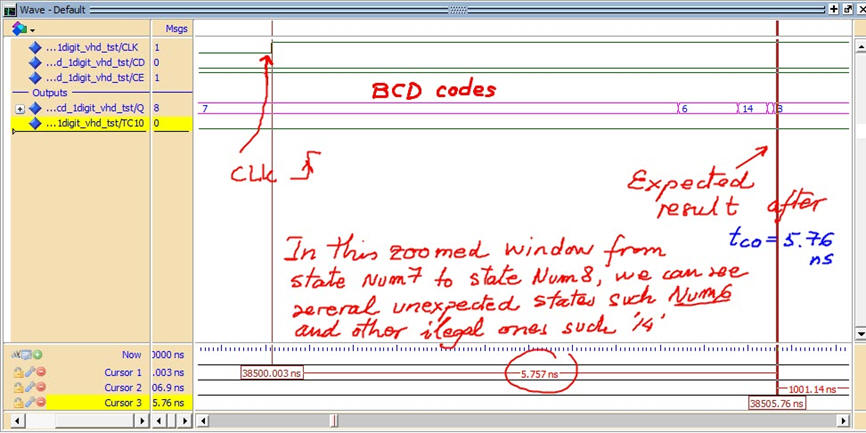Fig. 15. In this picture, several illegal codes are generated due different propagation times.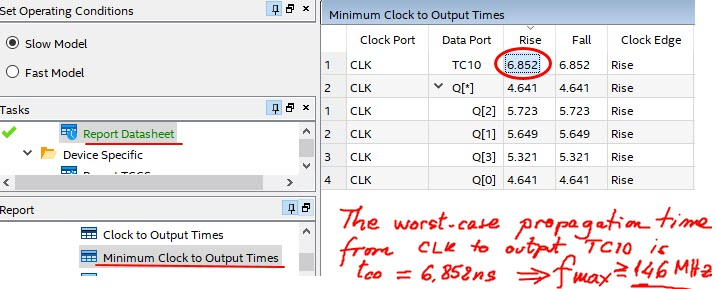Fig. 16. The worst-case scenario, the maximum operating frequency is calculated using the timing analyser tool.

5. Report

Project report starting with the template sheets of paper, scanned figures, file listings, docx , pptx, or any other resources

6. Prototyping

Use training boards and perform laboratory measurements to verify how the circuit works.